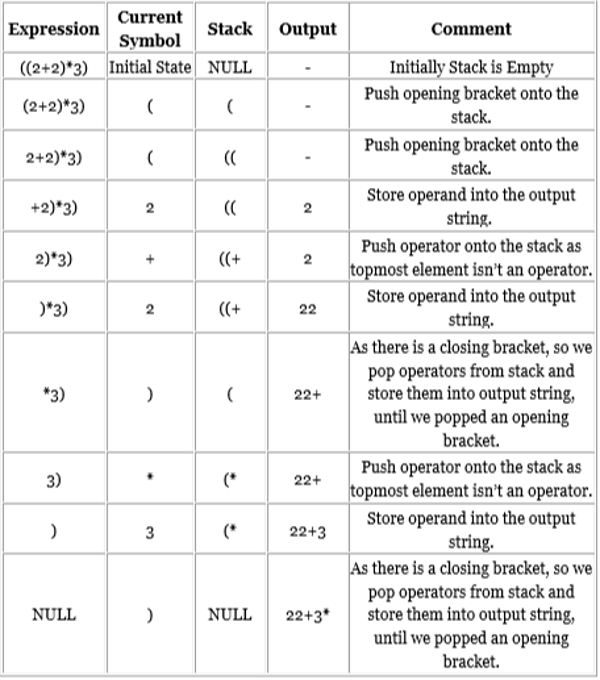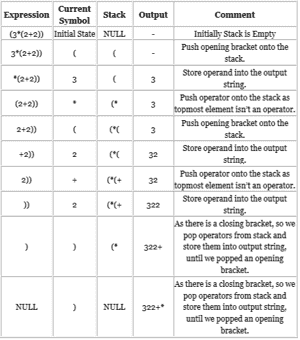Related Tags

postfix
prefix

# How to convert infix notation into postfix and prefix notations

Let’s recall how we write a mathematical equation with basic arithmetical operations. Here is an example of a simple equation:

((2 + 2) * 3)

This is the form that we normally use to write equations. This notation is called infix notation.

Due to the involvement of brackets, computers do not use this notation. Instead, they use either postfix or prefix notation.

## Postfix notation

In postfix notation, the operands come before their operators. Below is the same equation in postfix notation:

2 2 + 3 *

For the conversion of infix notation into postfix notation, we use the stack data structure. These are the rules for conversion:

1. Start scanning from left to right.

2. If there is an operand, store it into the output string.

3. If there is an operator, there are three possible cases.

• If the stack is empty, push the operator onto the stack.

• If the operator at hand has more precedence than the topmost operator on the stack, push the at-hand operator onto the stack.

• If the operator at hand has less or equal precedence than the topmost operator on the stack, pop the operator from the stack and store it into the output string, then push the at-hand operator onto the stack.

4. If there is an opening bracket, push it onto the stack.

5. If there is a closing bracket, pop operators from the stack and store them into the output string until we popped an opening bracket.

Let’s convert the given infix notation into postfix notation.## Prefix notation

In prefix notation, the operators come before their operands. This is also called Polish notation, or Warsaw notation. Below is the same equation in prefix notation:

*+ 2 2 3

For the conversion of infix notation into postfix notation, we use the stack data structure. These are the rules for conversion:

• Reverse the given infix expression.
• Change every ( to ) and every ) to (.
• Convert the expression into postfix notation.
• Reverse the resulting postfix expression.

Let’s convert the given infix notation into prefix notation.

Infix: ((2+2)*3)

Step 1: Reverse the expression.

)3*)2+2((

Step 2: Change every ‘(’ to ‘)’ and every ‘)’ to ‘(’.

(3*(2+2))

Step 3: Convert the expression into postfix.

Now, the resulting postfix expression is 322+*.

Step 4: Reverse the postfix expression.

*+ 2 2 3

This is the resulting prefix expression.RELATED TAGS

postfix
prefix

CONTRIBUTOR